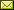## A Probabilistic Temporal Logic with Frequency Operators and Its Model Checking

 Takashi Tomita (Tokyo Institute of Technology) Shigeki Hagihara (Tokyo Institute of Technology) Naoki Yonezaki (Tokyo Institute of Technology)

 Probabilistic Computation Tree Logic (PCTL) and Continuous Stochastic Logic (CSL) are often used to describe specifications of probabilistic properties for discrete time and continuous time, respectively. In PCTL and CSL, the possibility of executions satisfying some temporal properties can be quantitatively represented by the probabilistic extension of the path quantifiers in their basic Computation Tree Logic (CTL), however, path formulae of them are expressed via the same operators in CTL. For this reason, both of them cannot represent formulae with quantitative temporal properties, such as those of the form ``some properties hold to more than 80% of time points (in a certain bounded interval) on the path.'' In this paper, we introduce a new temporal operator which expressed the notion of frequency of events, and define probabilistic frequency temporal logic (PFTL) based on CTL∗. As a result, we can easily represent the temporal properties of behavior in probabilistic systems. However, it is difficult to develop a model checker for the full PFTL, due to rich expressiveness. Accordingly, we develop a model-checking algorithm for the CTL-like fragment of PFTL against finite-state Markov chains, and an approximate model-checking algorithm for the bounded Linear Temporal Logic (LTL) -like fragment of PFTL against countable-state Markov chains.

In Fang Yu and Chao Wang: Proceedings 13th International Workshop on Verification of Infinite-State Systems (INFINITY 2011), Taipei, Taiwan, 10th October 2011, Electronic Proceedings in Theoretical Computer Science 73, pp. 79–93.
Published: 11th November 2011.

 ArXived at: https://dx.doi.org/10.4204/EPTCS.73.9 bibtex PDF
References in reconstructed bibtex, XML and HTML format (approximated).
 Comments and questions to:eptcs@eptcs.org For website issues:webmaster@eptcs.org